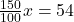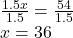## In a mathematics​ class 54, students received an A on the third​ test, which is ​150% of the students who received an A on the second test.

Question

In a mathematics​ class 54, students received an A on the third​ test, which is ​150% of the students who received an A on the second test. How many students received an A on the second​ test?

10,1 #16

in progress 0
6 months 2021-08-25T08:01:54+00:00 1 Answers 3 views 0

Number of students who received A grade on the second test = 36

Step-by-step explanation:

Given that:

Number of students who received A grade on third test = 54

This is 150% of the students who received A on the second test.

Let,

x be the number of students who received A on the second test.

150% of x = 541.5x=54

Dividing both sides by 1.5Hence,

Number of students who received A grade on the second test = 36